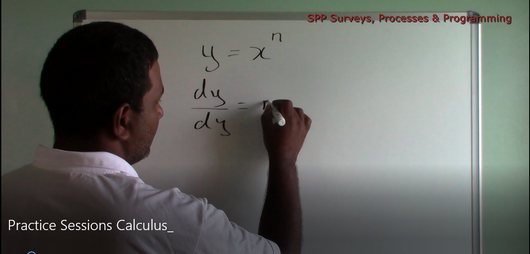# Calculus - Differentiation (Working Examples 2)

Regular price \$2.00 Sale

This 10:53 min tutorial is conducted by A. Deally, MBA, BSc. This tutorial focuses on Differentiation. Several examples are worked on in this video.

The examples worked in this video include:

1) y = x ^5

2) y = 3x ^4

3) y = 2x ^3 + 3x

4) y = 2x ^1/4

5) y = 2 √x

6) y = 1 / x^2

7) y = 1 / 2√x